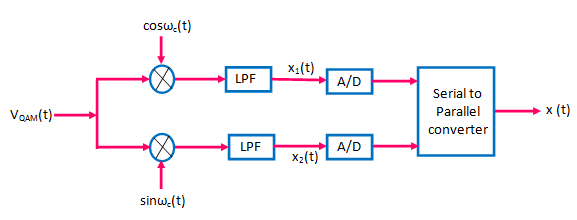The quadrature amplitude modulation (QAM) is similar to DSB-SC. The only difference is that QAM sends two message signals over the same spectrum .

Let the two message signals be x1(t) and x2(t) .

One of them is sent in phase i.e. by multiplying it with cos ωct and the other one x2(t) is sent in quadrature by multiplying it with sinωct .

Finally the two signals are added to obtain the QAM signals as shown in fig.1 .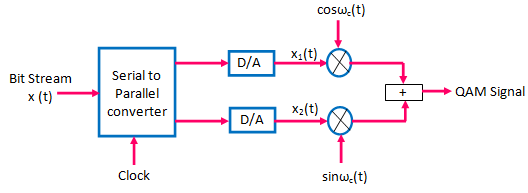Fig.1 : Generation of QAM Signal

Hence, the QAM signal is mathematically represented as below :

VQAM(t) = x1(t) cos ωct + x2(t) sinωct

The coherent detection is used at the receiver to recover the signal x(t) back .

The QAM signal at the receiver input is multiplied by locally generated carrier and the product is passed through a low pass filter .

If we multiply VQAM(t) by cos ωct, then we can recover x1(t) whereas multiplying VQAM(t) by sinωct can recover  x2(t) as under :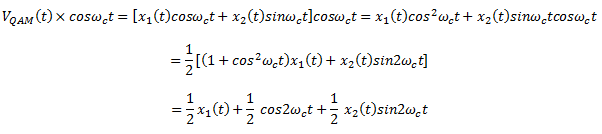If we pass this signal through a LPF, then, only the first term will be passed through to the output .

Therefore,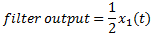Thus, we recover x1(t) if  VQAM(t) multiplied with cosωc(t) .

Similarly, if sinωc(t)  is multiplied with VQAM(t) then, we get,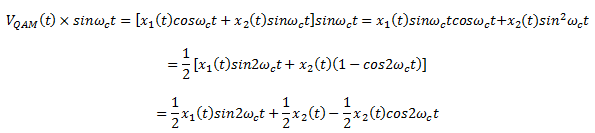If this is passed through a LPF, only the second term will be allowed to pass through .

Hence,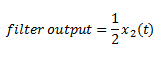Thus, we recover x2(t), if VQAM(t) is multiplied with  sinωct .

Fig.2 shows the block diagram of QAM receiver. QAM performs like SSB . The QAM system is easy to install .